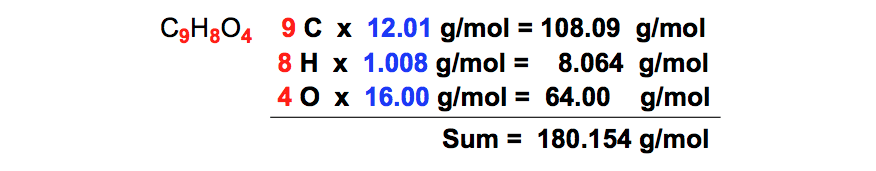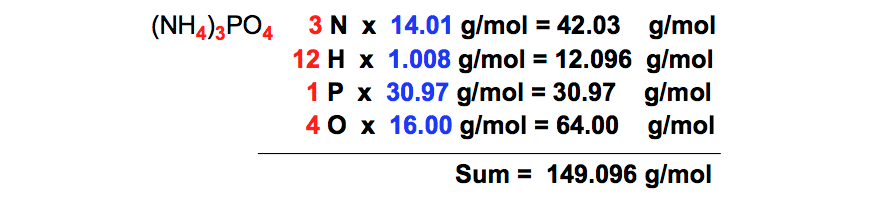Clutch Prep is now a part of Pearson
Ch.2 - Atoms & ElementsWorksheetSee all chapters

# Calculating Molar Mass (IGNORE)

See all sections
Sections
The Atom
Subatomic Particles
Isotopes
Ions
Atomic Mass
Periodic Table: Classifications
Periodic Table: Group Names
Periodic Table: Representative Elements & Transition Metals
Periodic Table: Element Symbols
Periodic Table: Elemental Forms
Periodic Table: Phases
Periodic Table: Charges
Calculating Molar Mass
Mole Concept
Law of Conservation of Mass
Law of Definite Proportions
Atomic Theory
Law of Multiple Proportions
Millikan Oil Drop Experiment
Rutherford Gold Foil ExperimentJules Bruno

Molar mass or molecular weight is a physical property that represents the mass of a substance divided by the amount of that substance. The common units are typically in grams per mole (g/mol), while the si units are in kilograms per mole (kg/mol).

How to Calculate Molar Mass

In order to determine the molar mass of a compound we must determine the number of atoms for the elements within a given compound.

For example, if we are asked to determine the molar mass of glucose, C6H12O6, we can solve with steps shown below.

STEP 1: Count the number of each element within the compound to get 6 carbons, 12 hydrogens and 6 oxygens.Number of atoms

STEP 2: Find the atomic mass for each chemical element from the periodic table.Periodic Table (Atomic Masses)

STEP 3: Multiply the number of each element by their atomic masses taken from the periodic table.Addition of Atomic Masses

STEP 4: Add up all the masses you got from multiplication together to give the overall molar mass of the compound.Molar Mass of Glucose

From simple compounds such as CO2, H2O, or ammonia to more complex compounds such as proteins, amino acids, acetone or acetic acid we now calculate their molar masses.

PRACTICE 1: Calculate the formula weight of aspirin, C9H8O4, based on the given molecular formula.Molar Mass (Aspirin)

Aspirin contains 9 carbon, 8 hydrogen and 4 oxygen atoms with a total mass of 180.154 g/mol.

PRACTICE 2: Calculate the molar mass of silver nitrate, AgNO3.Molar Mass (Silver Nitrate)

Silver nitrate contains 1 silver, 1 nitrogen and 3 oxygen atoms with a total mass of 169.88 g/mol.

PRACTICE 3: Determine the molecular weight of ammonium phosphate, (NH4)3PO4Molar Mass (Ammonium Phosphate)

Ammonium phosphate contains 3 nitrogens, 12 hydrogens, 1 phophate and 4 oxygen atoms with a total mass of 149.096 g/mol.

Why is the molar mass so important?

Molar mass serves as the foundation for many calculations and ideas found in chemistry. It serves as the bridge that connects together concepts in stoichiometry such as mass conversions, moles, avogadro’s number (6.022 x 1023) or in dimensional analysis such as density, amu, and moles.

In addition we discuss the average molar mass or average molecular mass of compounds when we cover isotopes, the colligative properties such as boiling point elevation or freezing point depression, and the rates of gases through effusion and diffusion.Jules Bruno

Jules felt a void in his life after his English degree from Duke, so he started tutoring in 2007 and got a B.S. in Chemistry from FIU. He’s exceptionally skilled at making concepts dead simple and helping students in covalent bonds of knowledge.